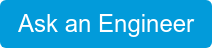×

# Humidity Definitions - Part 3

Parts Per Million by Weight (PPMw)
Parts per million by weight of dry gas (PPMw) is identical to PPMv except that the weight ratio changes with the molecular weight of the carrier gas.

Example 1.

If the frost point = -60°C and the carrier gas is hydrogen:Example 2.
If the frost point = -70°F and system total pressure is 14.7 PSIA, and the carrier gas is hydrogen:Molecular weight of common gases:Dew Point/Frost Point Relationships (FPR)
Below 0°C (32°F), dew point hygrometers measure the frost point temperature rather than the dew point. The tables below permit conversion from dew to frost point. For a more accurate conversion, consult Table 102 of the Smithsonian Meteorological Table.REFERENCE: Smithsonian Meteorological Tables, Sixth Revised Edition, List, Robert J., Publication No. 4014, Smithsonian Institution, Washington, D.C.

Pressure Conversion (PC)
As the total pressure of a gas sample changes, the partial pressures of the gases comprising the total pressure change in the same ratio.

Example 1.
If frost point = -60°C and the system total pressure is 1013 mb what is the frost point at 21 bar?From the Vapor Pressure Tables (over ice), the new Frost Point = -35.2°C.

Example 2.
If the frost point = -70°F and the system total pressure is 14.7 PSIA, what is the frost point at 84.7 PSIA?From the Vapor Pressure Tables (over ice) the new frost point = -44.5°F.

Rule of Thumb: If you increase the total pressure of a system, the dew/frost point temperature will increase. If you decrease the total pressure of a system, the dew/frost point temperature will decrease.